Manufacturing problems

Chapter 12 Class 12 Linear Programming
Concept wise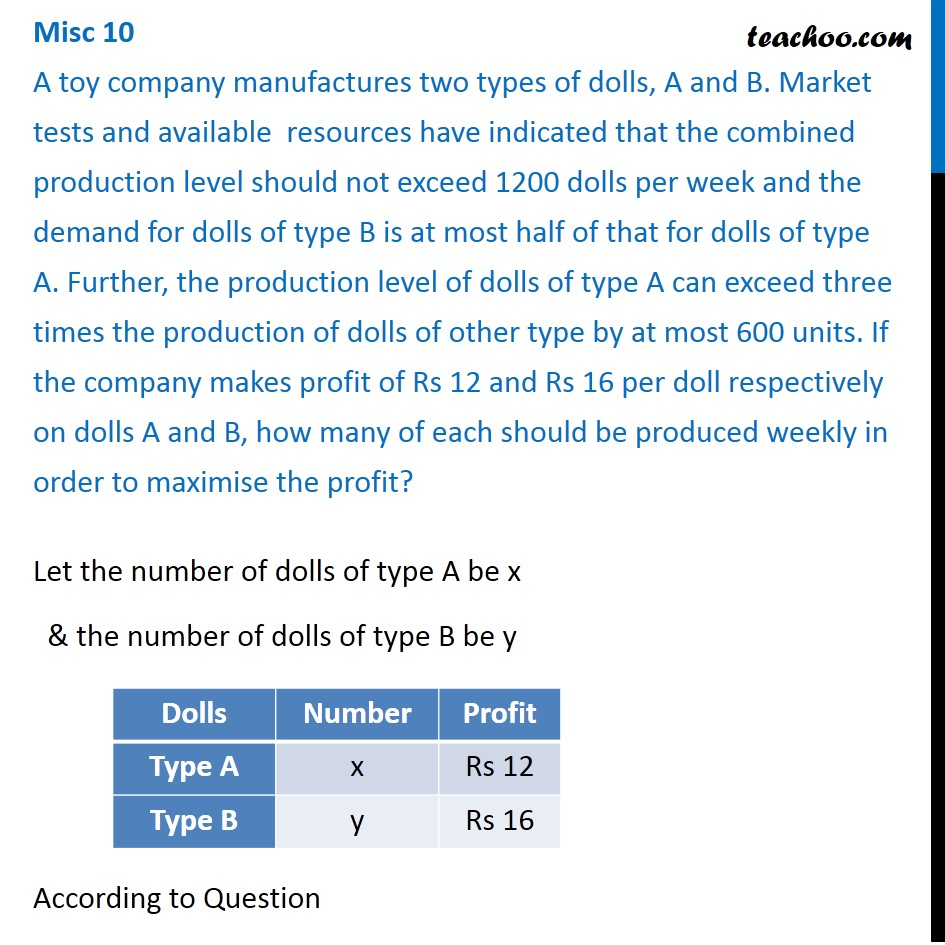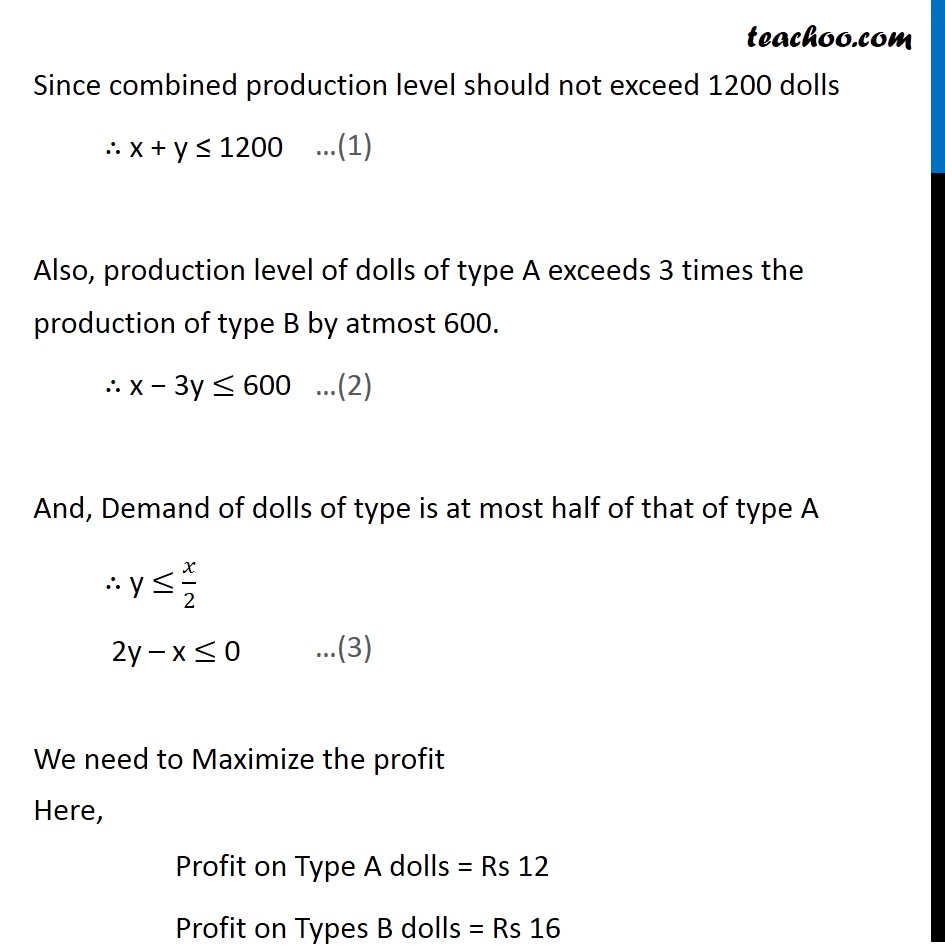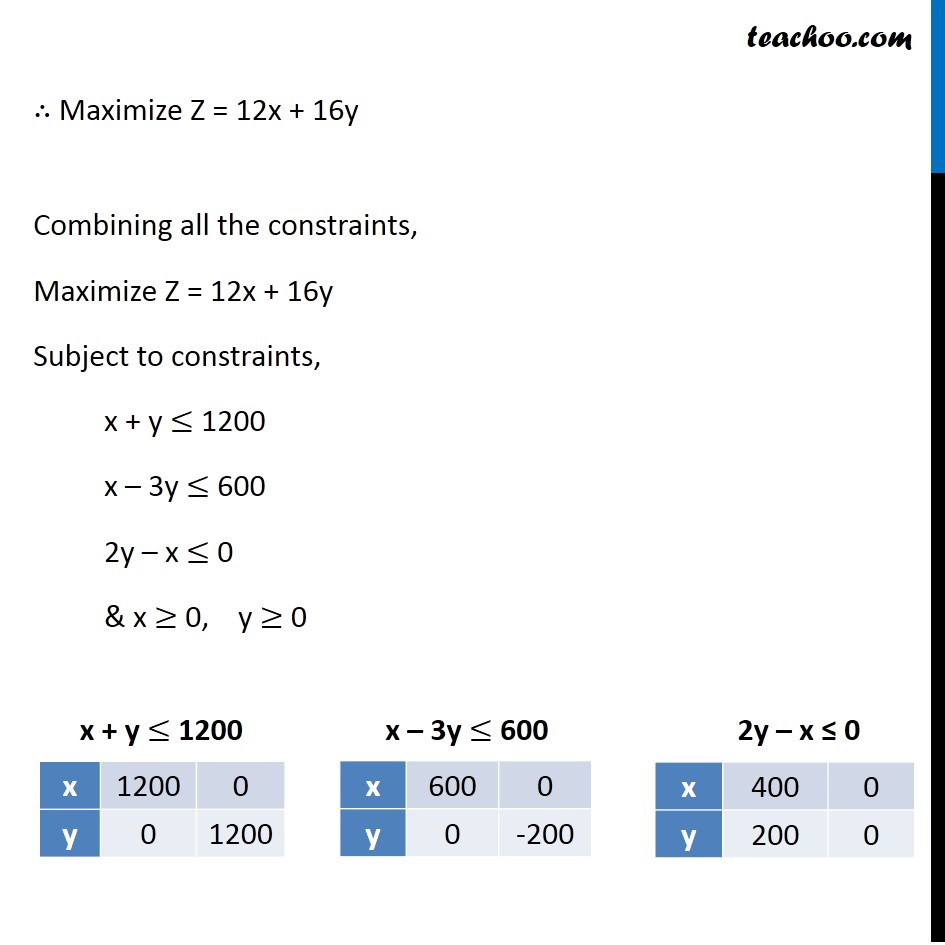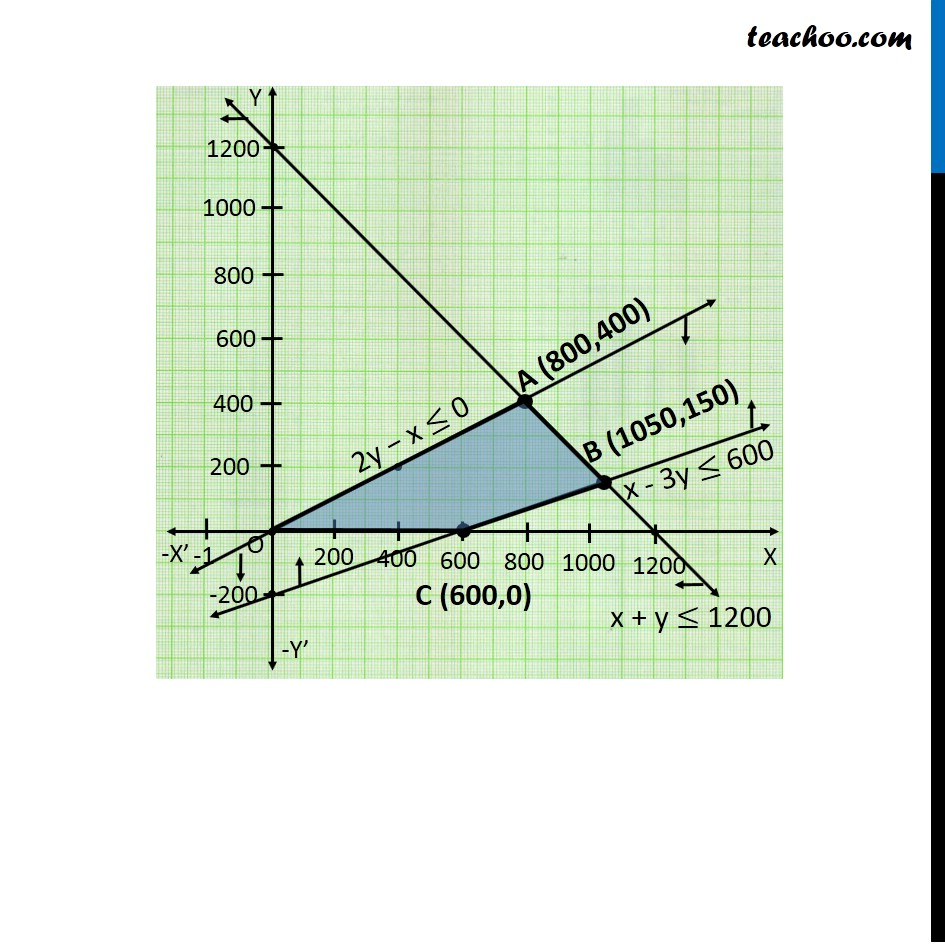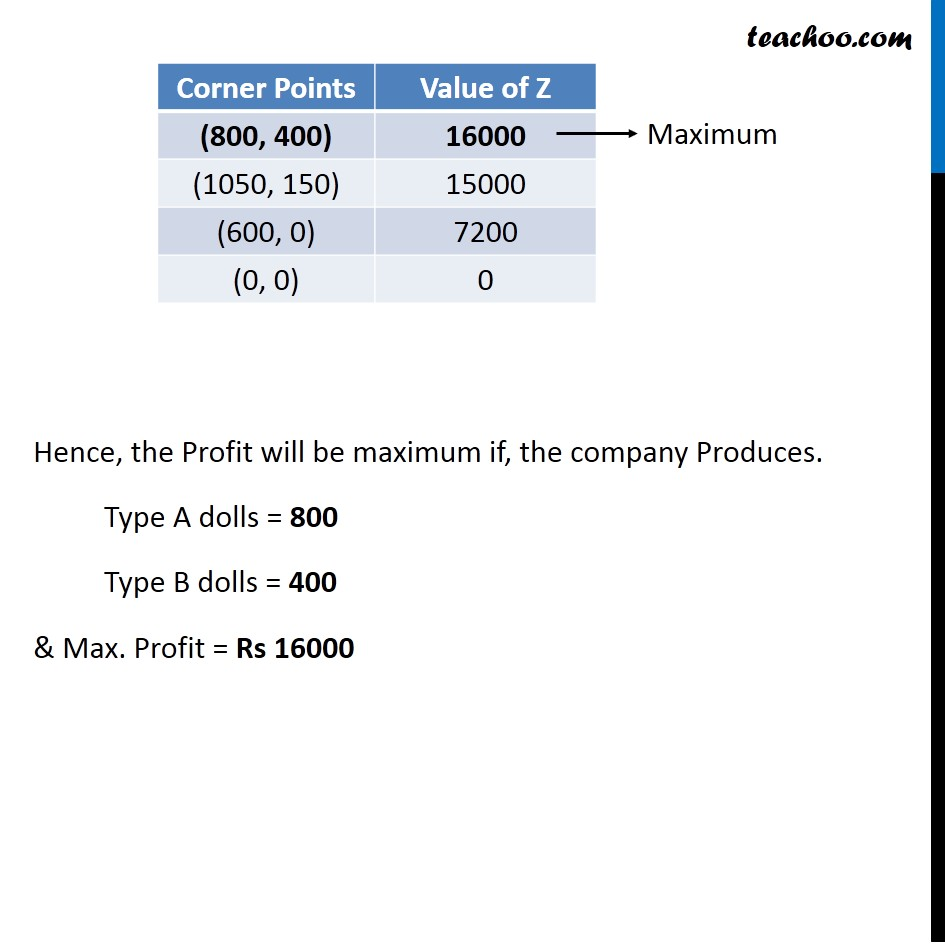Learn in your speed, with individual attention - Teachoo Maths 1-on-1 Class

### Transcript

Misc 10 A toy company manufactures two types of dolls, A and B. Market tests and available resources have indicated that the combined production level should not exceed 1200 dolls per week and the demand for dolls of type B is at most half of that for dolls of type A. Further, the production level of dolls of type A can exceed three times the production of dolls of other type by at most 600 units. If the company makes profit of Rs 12 and Rs 16 per doll respectively on dolls A and B, how many of each should be produced weekly in order to maximise the profit? Let the number of dolls of type A be x & the number of dolls of type B be y According to Question Since combined production level should not exceed 1200 dolls ∴ x + y ≤ 1200 Also, production level of dolls of type A exceeds 3 times the production of type B by atmost 600. ∴ x − 3y ≤ 600 And, Demand of dolls of type is at most half of that of type A ∴ y ≤ 𝑥/2 2y – x ≤ 0 We need to Maximize the profit Here, Profit on Type A dolls = Rs 12 Profit on Types B dolls = Rs 16 ∴ Maximize Z = 12x + 16y Combining all the constraints, Maximize Z = 12x + 16y Subject to constraints, x + y ≤ 1200 x – 3y ≤ 600 2y – x ≤ 0 & x ≥ 0, y ≥ 0 Hence, the Profit will be maximum if, the company Produces. Type A dolls = 800 Type B dolls = 400 & Max. Profit = Rs 16000